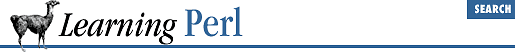home | O'Reilly's CD bookshelfs | FreeBSD | Linux | Cisco | Cisco Exam

### A.18 Chapter 19, CGI Programming

1. Here's one way to do it:

```use strict;
use CGI qw(:standard);

if(param()) {
my \$n1 = param('field1');
my \$n2 = param('field2');
my \$n3 = \$n2 + \$n1;
print p("\$n1 + \$n2 = <strong>\$n3</strong>\n");
} else {
print hr(), start_form();
print p("First Number:", textfield("field1"));
print p("Second Number:", textfield("field2"));
print end_form(), hr();
}
print end_html();```

If there's no input, simply generate a form with two textfields (using the ``` textfield``` method). If there is input, we add the two fields together and print the result.

2. Here's one way to do it:

```use strict;
use CGI qw(:standard);

print h1("Browser Detective"), hr();
my \$browser = \$ENV{'HTTP_USER_AGENT'};
\$_ = \$browser;

BROWSER:{
if (/msie/i) {
msie(\$_);
} elsif (/mozilla/i) {
netscape(\$_);
} elsif (/lynx/i) {
lynx(\$_);
} else {
default(\$_);
}
}

print end_html();
sub msie{
print p("Internet Explorer: @_.  Good Choice\n");
}

sub netscape {
print p("Netscape: @_.  Good Choice\n");
}

sub lynx {
print p("Lynx: @_.  Shudder...");
}

sub default {
print p("What the heck is a @_?");
}```

The key here is checking the environment for the HTTP_USER_AGENT variable. Although this isn't implemented by every server, many of them do set it. This is a good way to generate content geared to the features of a particular browser. Note that we're just doing some basic string matching (case insensitive) to see what they're using (nothing too fancy).A.17 Chapter 18, Converting Other Languages to PerlB. Libraries and Modules